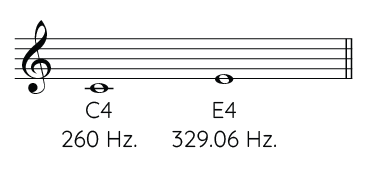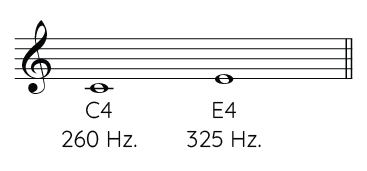#### Comparing intervals using the mathematical ratio

We are going to use the mathematical ratio to compare two major thirds. For the first third, we will use Pythagorean tuning and for the other just tuning (see this article for information on different tuning systems). In the following table, we see the frequencies of the notes and calculate the mathematical ratio of each interval:

Temperament C4 E4 Mathematical ratio
Major third
Pitagorean tuning
260 329.06 329.06 / 260 = 1.2656153846Major third
Just intonation
260 325 325 / 260 = 1.25The just intonation's major third is smaller than the Pythagorean tuning one (1.25 is less than 1.2656153846). However, the numbers do not help to discern the difference in size. How small or large is the difference? How evident is the difference? The use of cents will facilitate comparison.